### Machine learning-aided CFD with OpenFOAM and PyTorch

Andre Weiner
TU Braunschweig, ISM, Flow Modeling and Control GroupThese slides and most of the linked resources are licensed under a

## Outline

1. ML and CFD in a nutshell
2. Applications
1. Validating a boundary layer model for species
2. Detecting coherent structures in transonic buffets
3. Active control of the flow past a cylinder
3. (Random) lessons learned

## Its a training ...

Feel free to ask questions at any time!

## Why combine ML and CFD?

Computational fluid dynamics (CFD)

• produces vast amounts of data
• requires data

Machine learning (ML)

• finds patterns in data
• creates representations of data

## What data are we talking about?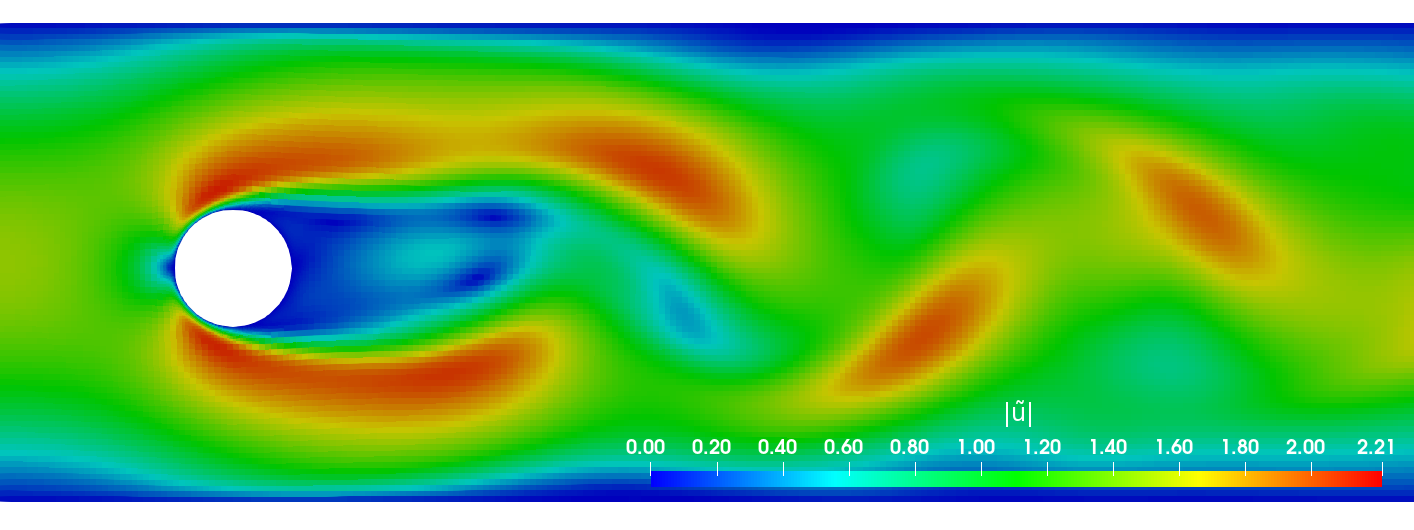Primary data: scalar/vector fields, boundary fields, integral values


# log.rhoPimpleFoam
Courant Number mean: 0.020065182 max: 0.77497916
deltaT = 6.4813615e-07
Time = 1.22219e-06

PIMPLE: iteration 1
diagonal:  Solving for rho, Initial residual = 0, Final residual = 0, No Iterations 0
DILUPBiCGStab:  Solving for Ux, Initial residual = 0.0034181127, Final residual = 6.0056507e-05, No Iterations 1
DILUPBiCGStab:  Solving for Uy, Initial residual = 0.0052004883, Final residual = 0.00012352706, No Iterations 1
DILUPBiCGStab:  Solving for e, Initial residual = 0.06200185, Final residual = 0.0014223046, No Iterations 1
limitTemperature limitT Lower limited 0 (0%) of cells
limitTemperature limitT Upper limited 0 (0%) of cells
limitTemperature limitT Unlimited Tmax 329.54945
Unlimited Tmin 280.90821


Checking geometry...
...
Mesh has 2 solution (non-empty) directions (1 1 0)
All edges aligned with or perpendicular to non-empty directions.
Boundary openness (1.4469362e-19 3.3639901e-21 -2.058499e-13) OK.
Max cell openness = 2.4668495e-16 OK.
Max aspect ratio = 3.0216602 OK.
Minimum face area = 7.0705331e-08. Maximum face area = 0.00033983685.  Face area magnitudes OK.
Min volume = 1.2975842e-10. Max volume = 6.2366859e-07.  Total volume = 0.0017254212.  Cell volumes OK.
Mesh non-orthogonality Max: 60.489216 average: 4.0292071
Non-orthogonality check OK.
Face pyramids OK.
Max skewness = 1.1453509 OK.
Coupled point location match (average 0) OK.



Secondary data: log files, input dictionaries, mesh quality metrics, ...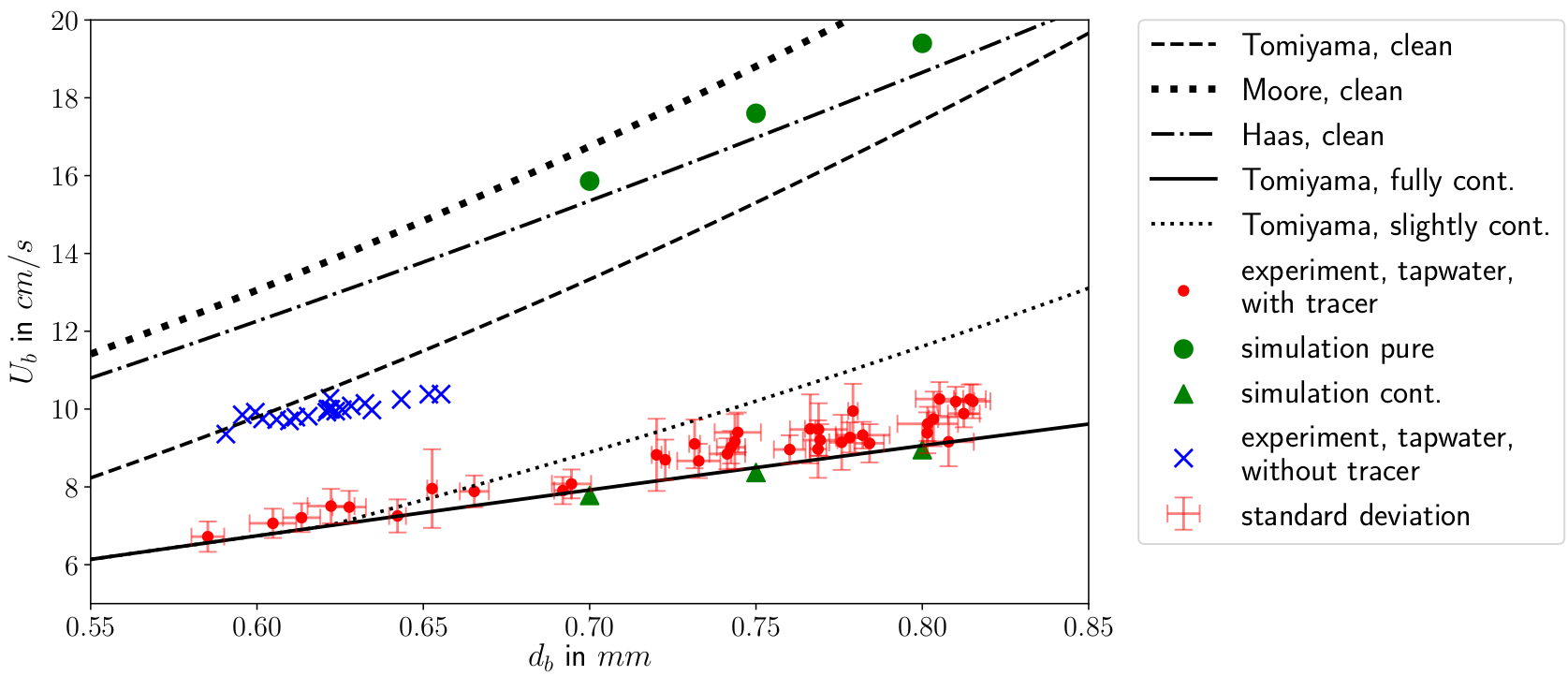The data do not necessarily have to come only from simulations.
Image Source

## How are ML, CFD, and data connected?Example: creating a surrogate or reduced-order model based on numerical data.Example: creating a space and time dependent boundary condition based on numerical or experimental data.Example: creating closure models based on numerical data.Example: active flow control or shape optimization.

## Types of ML

### Supervised learningCreating a mapping from features to labels based on examples.

## Unsupervised learningFinding patterns in unlabeled data.

## (Deep) Reinforcement learning

Deep reinforcement learning (DRL) solves high-dimensional optimization problems.

• active flow control
• shape optimization
• ...

## Application I:

Validating a boundary layer model for species transfer using a single-phase simulation approach

github.com/AndreWeiner/sgs_model_test_transient

requirements: Docker, datasets linked in repo

This is joint work with Claire Claassen, Irian Hierck, Hans Kuipers, and Maike Balthussen.

The Eindhoven University of Technology
Multi-Scale Modeling of Multi-Phase Flows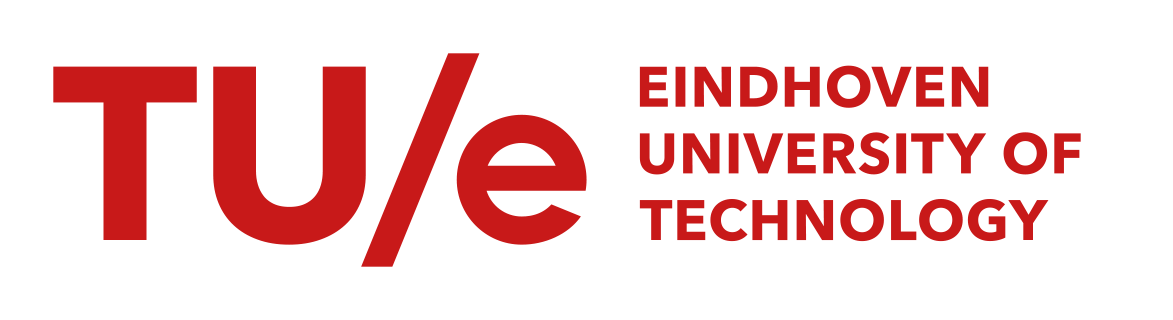## Gas-liquid reactors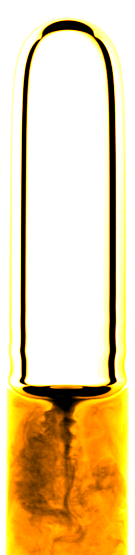micro reactor
size: millimeter
source: SPP 1740

prediction of

• mass transfer
• enhancement
• mixing
• conversion
• selectivity
• yield
• ...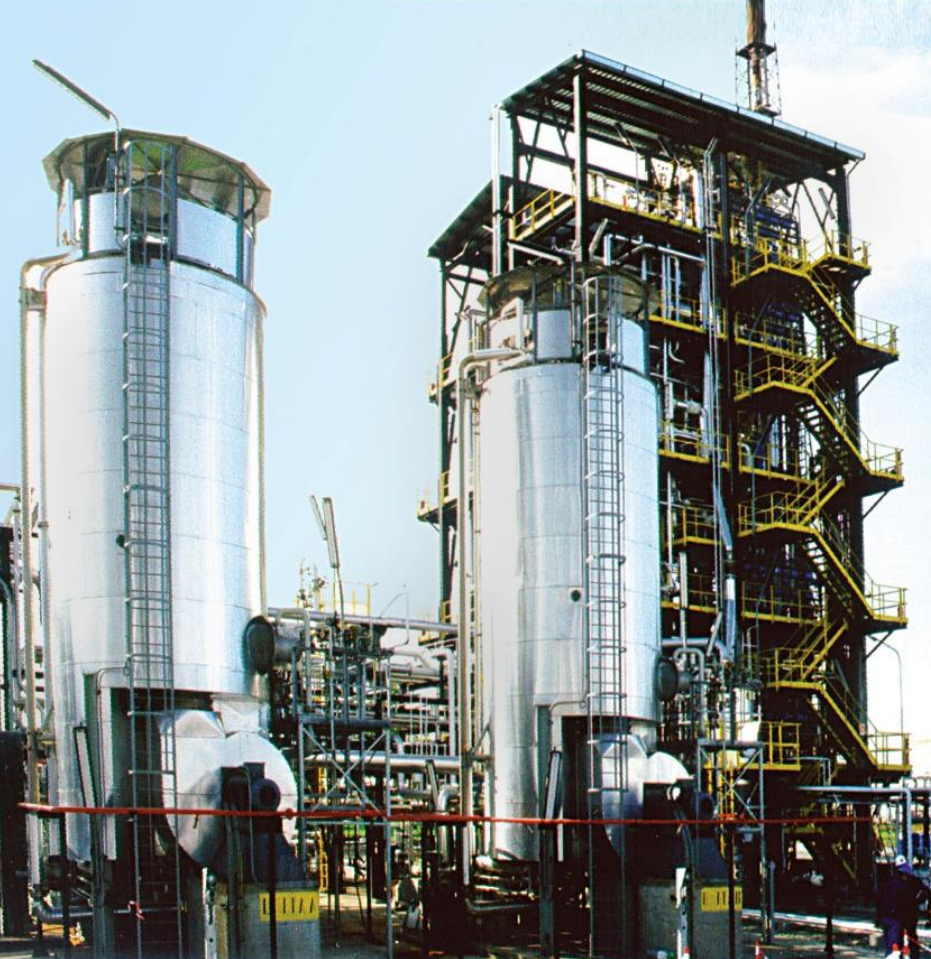bubble column reactor
size: meter
source: R. M. Raimundo, ENI

## Specimen calculation

$d_b=1~mm$ water/oxygen at room temperature

• $Pe = Sc\ Re = \nu_l / D_{O_2} \cdot U_b d_b/\nu_l \approx 10^5$
• $$Re\approx 250;\quad \delta_h/d_b \propto Re^{-1/2};\quad\delta_h\approx 45~\mu m$$
• $$Sc\approx 500;\quad \delta_c/\delta_h \propto Sc^{-1/2};\quad\delta_c\approx 2.5~\mu m$$

$\delta_h/\delta_c$ typically 10 ... 100

feasible simulations up to $Pe\approx 10000$ (3D, HPC)

solution:
boundary layer models (subgrid-scale models)

new problem:
How to validate the models?

solution:
ML-based single-phase simulation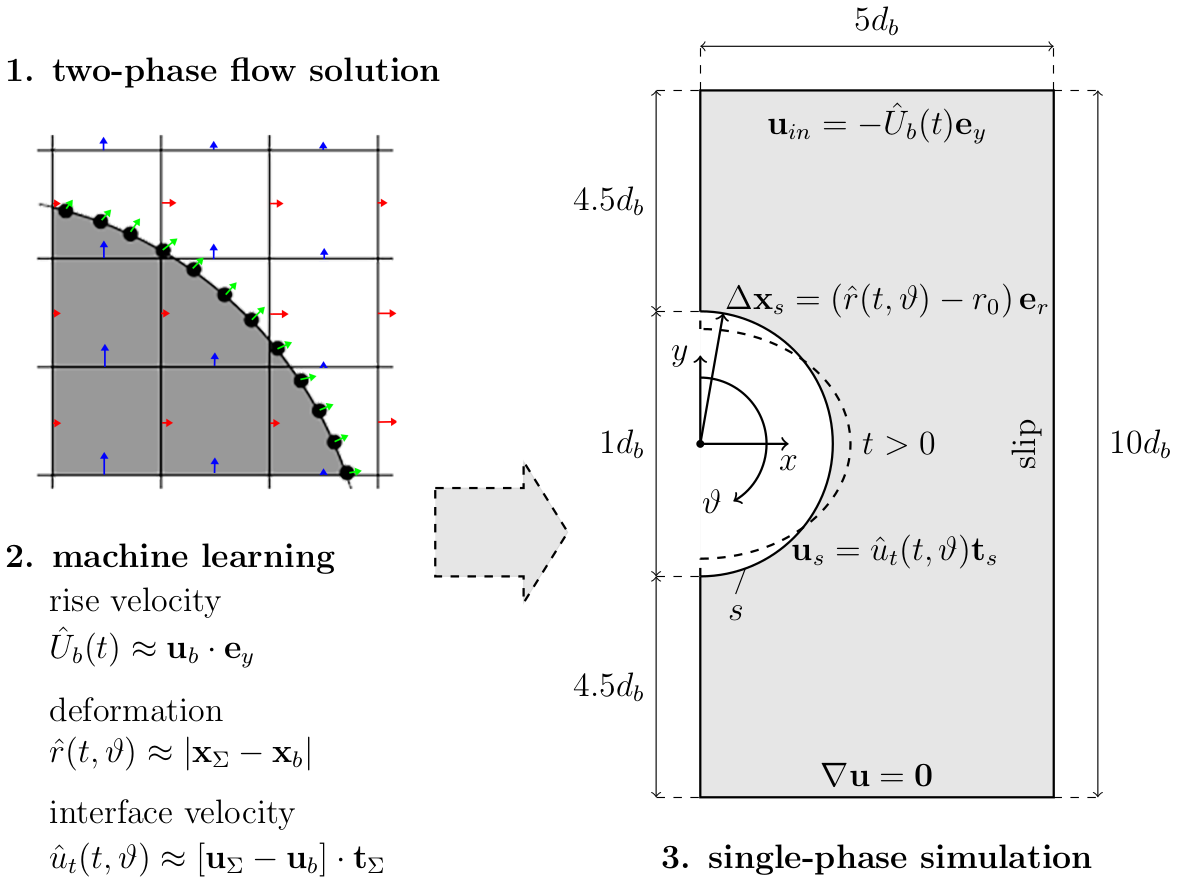Single-phase simulation approach; preprint will be linked soon in the repository.

Implementation

1. extract data from two-phase simulation
2. process and visualize data (Numpy, Pandas, Matplotlib)
3. train ML models (multilayer preceptrons implemented in PyTorch)
4. export models to TorchScript
5. implement BCs in OpenFOAM and compile
6. load ML models and perform simulations

def __init__(self, model_dict, model_path, axes_model, scaler_tt, scaler_rad):
# snip

def _inverse_scale(self, y):

axes = self._axes_model(X[:, 0].unsqueeze(-1))
# snip
return re

def forward(self, X):
X = self._scale(X)
X = self._inverse_scale(self._model(X)).squeeze()


Hide feature normalization and model assembly using a new module.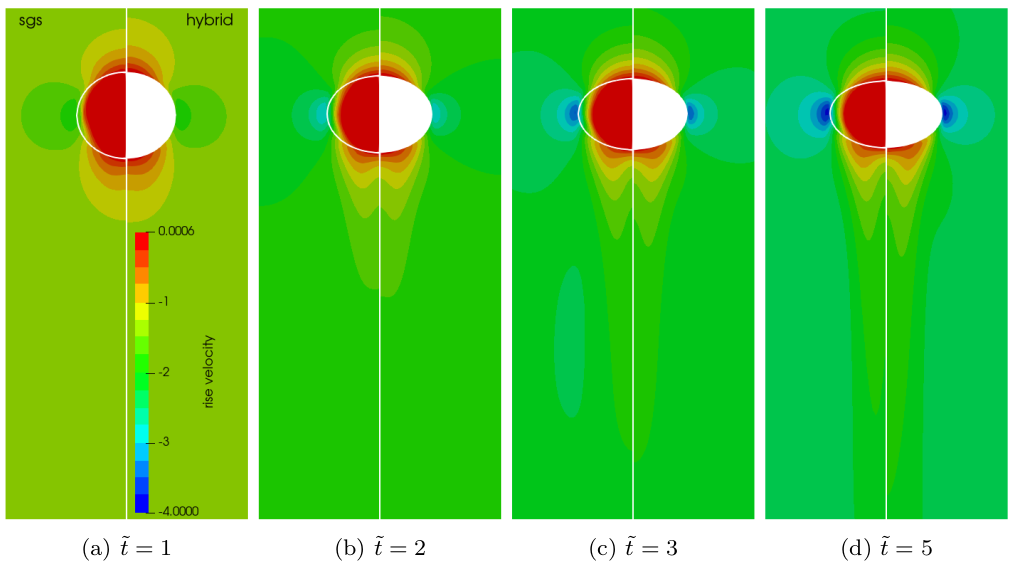Two-phase velocity field (left half) versus single-phase velocity field (right half); speed-up of 20-40x with 120x finer mesh at surface.

Transient concentration field for different Reynolds numbers $Re$ and constant Schmidt number $Sc=100$.

Mesh motion and zoom view of concentration boundary layer for $Re=569$ and $Sc=100$.Global Sherwood number $Sh$ for two different mesh resolutions (3250 and 6500 cells/diameter). ~7h, serial, 2.4 GHz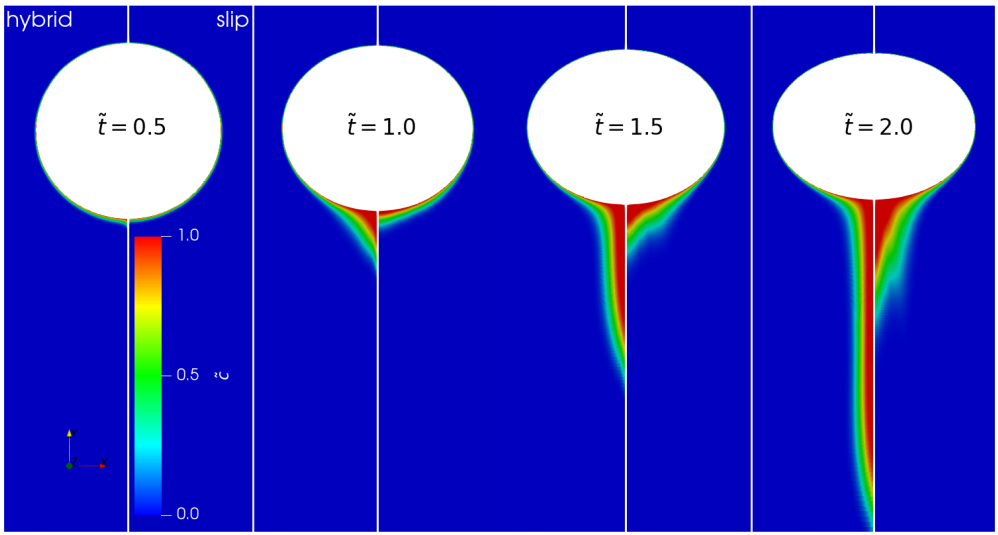Full hybrid approach (left half) vs. free-slip BC for surface velocity (right half).

## Application II:

Finding coherent structures in transonic shock buffets at a NACA-0012 airfoil

github.com/AndreWeiner/flowtorch

requirements: flowTorch, Jupyter lab, surface data

dynamic access (dmd_naca0012_surface.ipynb)


git clone https://github.com/AndreWeiner/flowtorch.git
python3 setup.py bdist_wheel
pip3 install dist/flowTorch-0.1-py3-none-any.whl
cd docs/source/notebooks/
jupyter lab


static access (flowTorch HTML docs)

2. unzip
3. open index.html in web browser
4. navigate to DMD analysis of airfoil surface data

This is joint work with Richard Semaan (group leader) within the FOR 2895.

Technische Universität Braunschweig
Flow Modeling and Control Group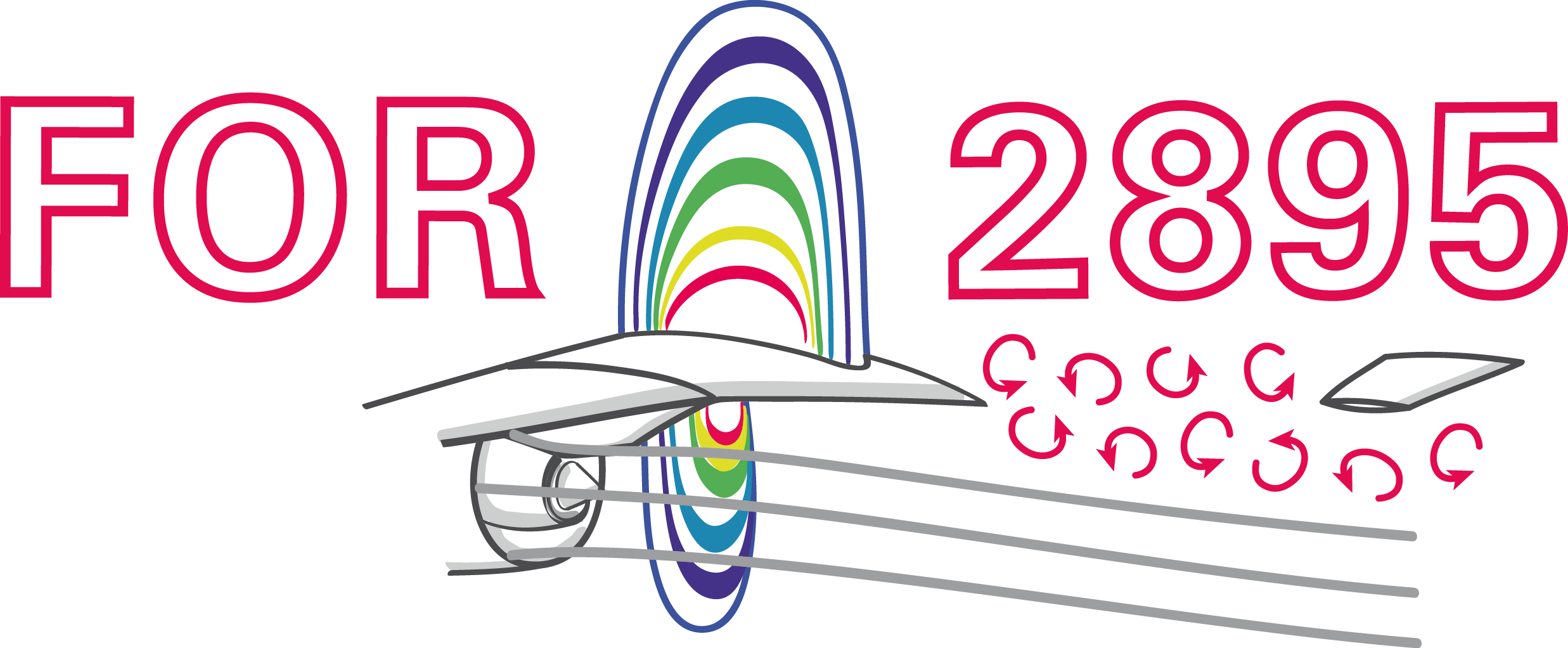Transonic buffet on a NACA-0012 airfoil at Reynolds number $Re=10^7$, Mach number $Ma=0.75$, and angle of attack $\alpha=4^\circ$.

## dynamic mode decomposition (DMD)## DMD in a nutshell

 1 $\mathbf{X}$, $\mathbf{X}^\prime$ data matrices; snapshots form colum vectors 2 $\mathbf{X} = \mathbf{U}\mathbf{\Sigma}\mathbf{V}^\dagger$ singular value dec. 3 $\mathbf{A} = \mathbf{U}^\dagger_r\mathbf{X}^\prime\mathbf{V}_r\mathbf{\Sigma}^{-1}_r$ best-fit linear operator 4 $\mathbf{AW} = \mathbf{W\Lambda}$ eigen value problem 5 $\mathbf{\Phi} = \mathbf{X}^\prime \mathbf{V\Sigma}^{-1}\mathbf{W}$ DMD modes

Refer to the notebook Introduction to DMD for details.DMD modes (columns of $\mathbf{\Phi}$)

characteristic solution, time dynamics

$$\mathbf{x}(t) = \mathbf{\Phi} e^{\mathbf{\Omega}t}\mathbf{\Phi}^{-1}\mathbf{x}_0\text{,}\quad \mathbf{\Omega} := \mathrm{log}(\mathbf{\Lambda})/\Delta t$$

growth rates, frequencies

$$a_i = Re(\omega_i)\text{,}\quad 2\pi f_i = Im(\omega_i)$$

Implementation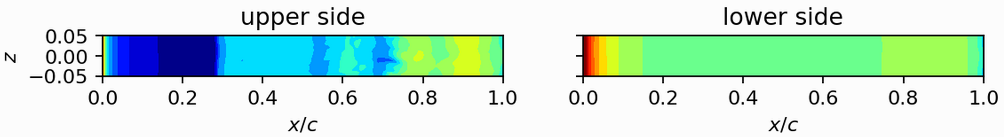1. load surface pressure and assemble data matrix
2. compute SVD and analyze singular values
3. compute DMD using flowtorch.analysis.dmd.DMD
4. analyze frequencies and DMD modes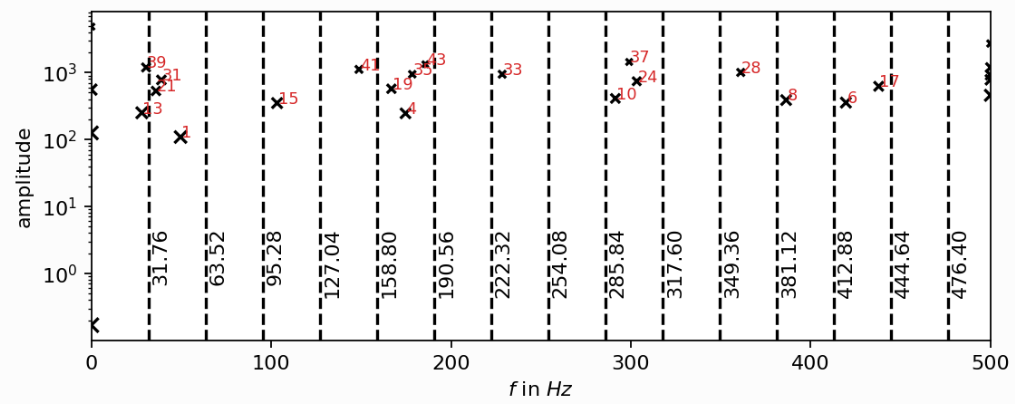Frequency spectrum; the experimental buffet frequency is $f\approx 31Hz$; source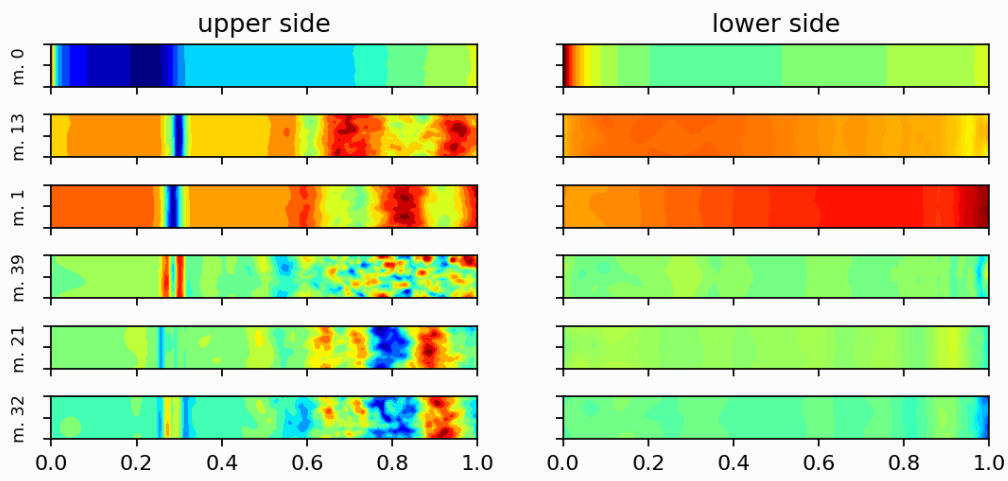DMD modes associated with the buffet frequency and steady mode (top).


import torch as pt

# select a subset of the available snapshots
window_times = [time for time in times if float(time) >= 4.0]

# assemble the data matrix
for i, time in enumerate(window_times):
# load the vorticity vector field, take the z-component [:, 2], and apply the mask


## Application III:

Active control of the flow past a cylinder by rotating the cylinder

github.com/darshan315/flow_past_cylinder_by_DRL

requirements: Singularity, HPC with SLURM scheduler (recommended)

The results were produced by Darshan Thummar during his student project (Studienarbeit) at the institute of fluid mechanics.Flow past a circular cylinder at $Re=100$.

## Can we stabilize the flow by rotating the cylinder?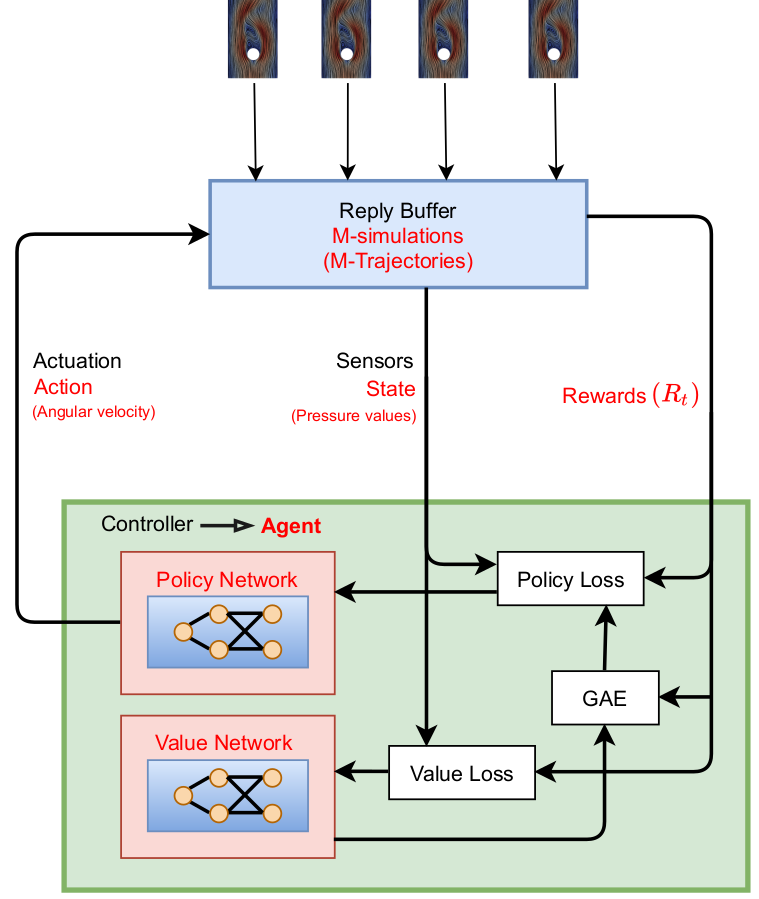Proximal policy optimization (PPO) workflow (GAE - generalized advantage estimate).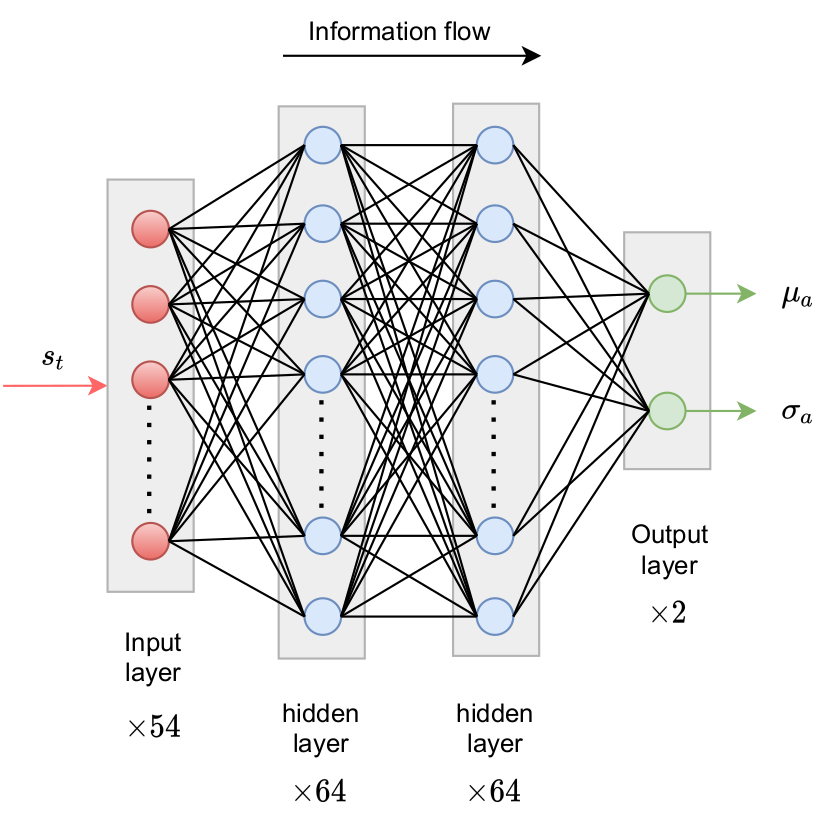Exploration by sampling next action from a Gaussian distribution.

reward at time $t$

$$R_t = r_0 - \left( r_1 c_D + r_2 c_L + r_3 \dot{\theta} + r_4 \ddot{\theta} \right)$$

• $c_D$ - drag coefficient
• $c_L$ - lift coefficient
• $\dot{\theta}$ - angular velocity
• $\ddot{\theta}$ - angular velocity
• $r_i$ - constants

implementation - Python/PyTorch

1. create policy and value networks
2. fill trajectory buffer (run simulations)
3. compute discounted rewards
4. update networks using policy and value loss
5. go back to 1. until converged

implementation - OpenFOAM

2. sample and apply action
3. write trajectory (state-action pairs)

Boundary condition defined in 0/U


cylinder
{
type            agentRotatingWallVelocity;
// center of cylinder
origin          (0.2 0.2 0.0);
// axis of rotation; normal to 2D domain
axis            (0 0 1);
// name of the policy network; must be a torchscript file
policy          "policy.pt";
// when to start controlling
startTime       0.01;
// how often to evaluate policy
interval        20;
// if true, the angular velocity is sampled from a Gaussian distribution
// if false, the mean value predicted by the policy is used
train           true;
// currently ignored
absOmegaMax     0.05;
}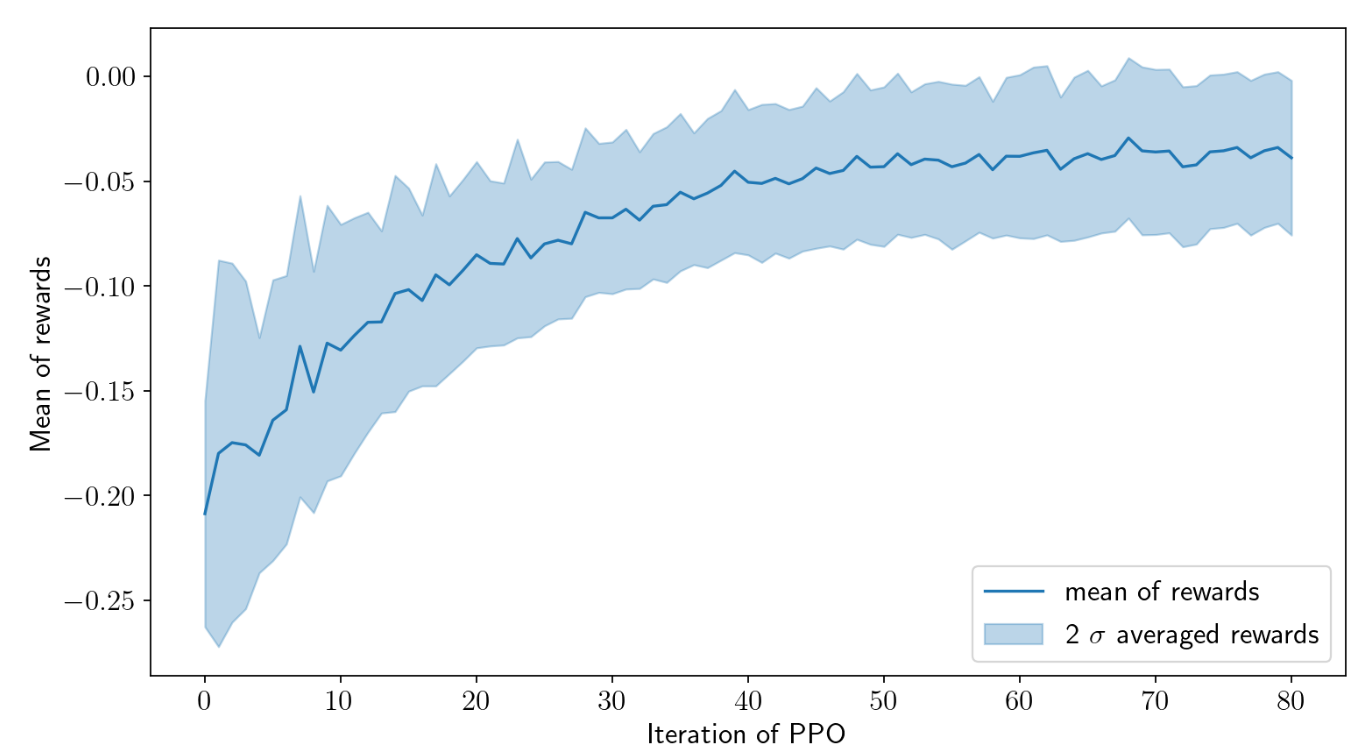Cumulative rewards plotted against PPO iterations.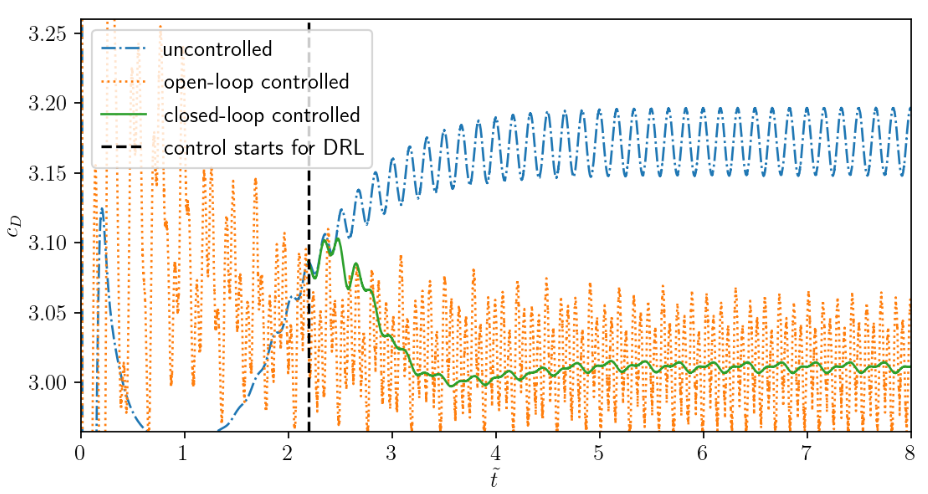Drag coefficient $c_D$ acting on cylinder.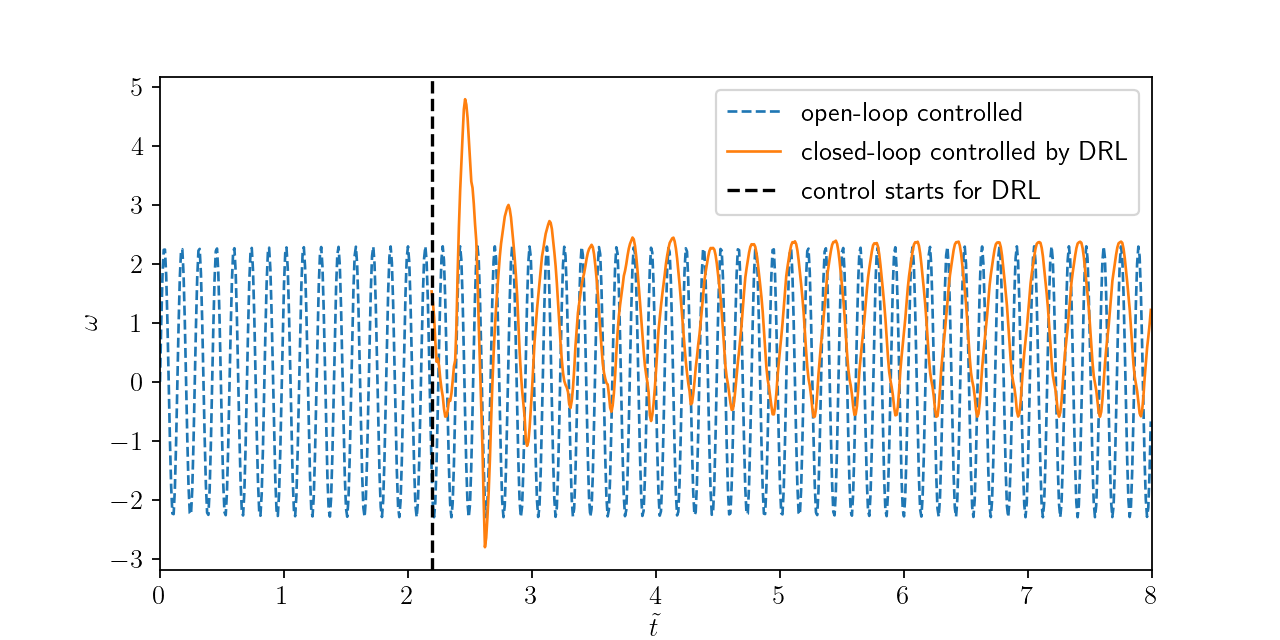Angular velocities of closed-loop and open-loop controllers.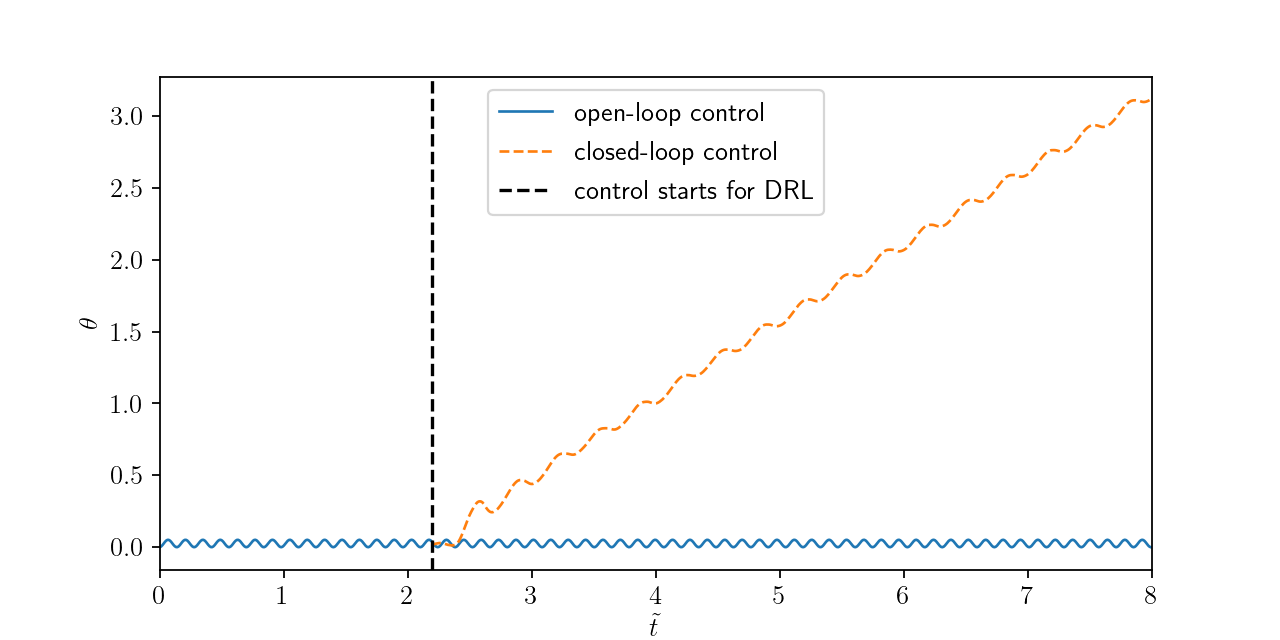Temporal evolution of polar angle.

## (Random) lessons learned

1. Finding good features of often hard

• use physical relations/intuition
• extract as many features as possible
• use automated feature selection
• make sure that the features are available in your target application!

2. Learn the mapping that you really need

classical wall function

1. take suitable function $y^+ = y^+(u^+)$ (e.g. Spalding)
2. iteratively find $u_\tau$ given $y_p$ and $u_p$
3. use $u_\tau$ to correct $\tau_w$ ($\nu_t$ in OpenFOAM)

data-driven wall function

1. collet boundary layer data
2. learn $\hat{y}^+\approx y^+(u^+)$ to replace analytical function
3. learn $\hat{\tau}_w \approx \tau_w(y_p, u_p)$ (apply typical normalization)

3. Remove as much variance from the data as possible before the training

• use physical relations, e.g, learn the deviation from an analytical solution rather than replacing it
• combine multiple models rather than creating a "master model"

4. (Most often) the exact ML algorithm is not all that important

• keep it as simple, e.g., use polynomial regression if you have few features and low nonlinearity
• create an automated pipeline to test a variety of models and hyper-parameters
• use what it easily available to you
• if you have no clue how hard the problem is, start with simple neural networks

5. (Most often) the network architecture is not all that important

• establish a suitable base line model
• use automated hyperparameter optimization
• build mathematical constraints into the architecture (e.g., boundedness and symmetries)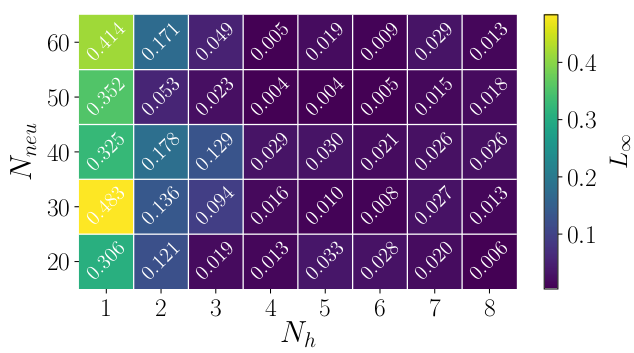Image source

6. Check multiple metrics during training

• $L_2$ or $R^2$ values often aren't meaningful
• check (at least) $L_\infty$
• examine the error distribution
• examine which features lead to large errors
Image source

7. Treat nonlinear optimization as an experiment!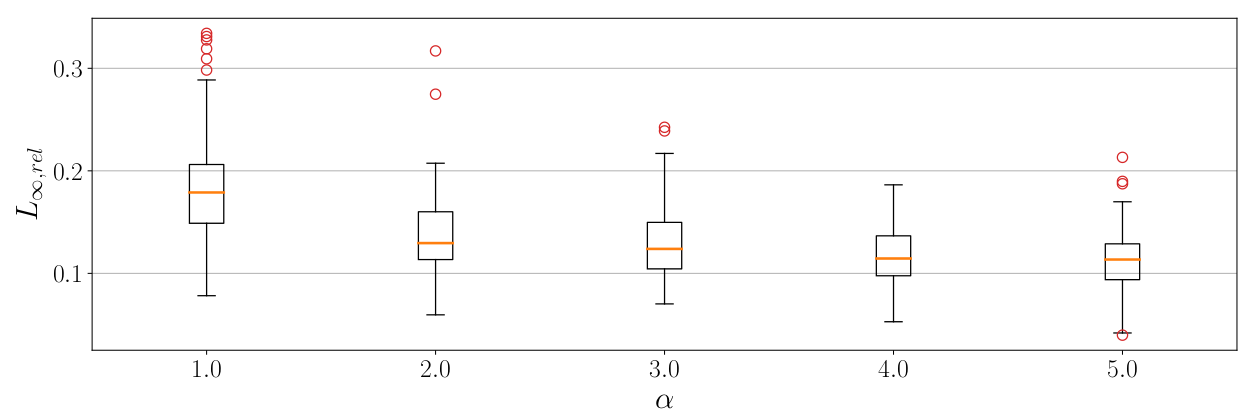Box plots are a great tool to visualize a repeated experiment; image source.

8. Use classical solution approaches rather than ML if available

# THE END

### Thank you for you attention!